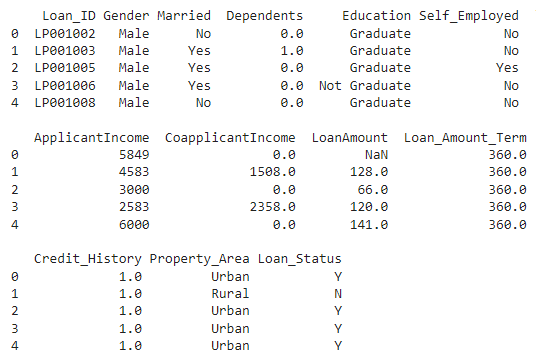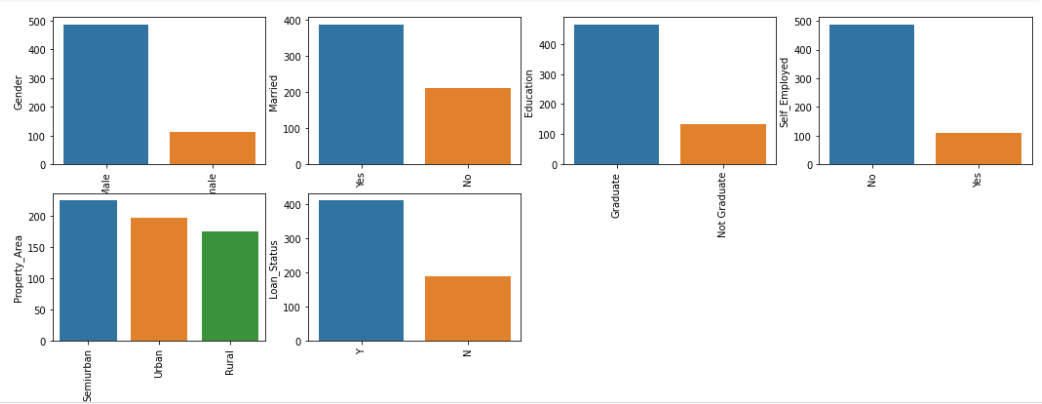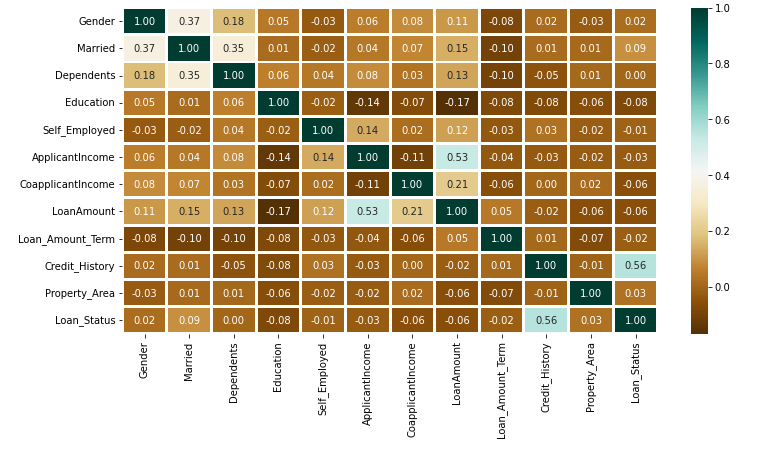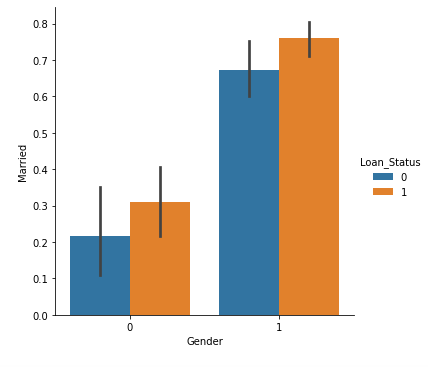Open in App
Not now

# Loan Approval Prediction using Machine Learning

• Last Updated : 23 Sep, 2022

LOANS are the major requirement of the modern world. By this only, Banks get a major part of the total profit. It is beneficial for students to manage their education and living expenses, and for people to buy any kind of luxury like houses, cars, etc.

But when it comes to deciding whether the applicant’s profile is relevant to be granted with loan or not. Banks have to look after many aspects.

So, here we will be using Machine Learning with Python to ease their work and predict whether the candidate’s profile is relevant or not using key features like Marital Status, Education, Applicant Income, Credit History, etc.

## Loan Approval Prediction using Machine Learning

The dataset contains 13 features :

## Importing Libraries and Dataset

Firstly we have to import libraries :

• Pandas – To load the Dataframe
• Matplotlib – To visualize the data features i.e. barplot
• Seaborn – To see the correlation between features using heatmap

## Python3

 `import` `pandas as pd ` `import` `numpy as np ` `import` `matplotlib.pyplot as plt ` `import` `seaborn as sns ` ` `  `data ``=` `pd.read_csv(``"LoanApprovalPrediction.csv"``) `

Once we imported the dataset, let’s view it using the below command.

## Python3

 `data.head(``5``)`

Output:## Data Preprocessing and Visualization

Get the number of columns of object datatype.

## Python3

 `obj ``=` `(data.dtypes ``=``=` `'object'``) ` `print``(``"Categorical variables:"``,``len``(``list``(obj[obj].index)))`

Output :

`Categorical variables: 7 `

As Loan_ID is completely unique and not correlated with any of the other column, So we will drop it using .drop() function.

## Python3

 `# Dropping Loan_ID column ` `data.drop([``'Loan_ID'``],axis``=``1``,inplace``=``True``)`

Visualize all the unique values in columns using barplot. This will simply show which value is dominating as per our dataset.

## Python3

 `obj ``=` `(data.dtypes ``=``=` `'object'``) ` `object_cols ``=` `list``(obj[obj].index) ` `plt.figure(figsize``=``(``18``,``36``)) ` `index ``=` `1` ` `  `for` `col ``in` `object_cols: ` `  ``y ``=` `data[col].value_counts() ` `  ``plt.subplot(``11``,``4``,index) ` `  ``plt.xticks(rotation``=``90``) ` `  ``sns.barplot(x``=``list``(y.index), y``=``y) ` `  ``index ``+``=``1`

Output:As all the categorical values are binary so we can use Label Encoder for all such columns and the values will change into int datatype.

## Python3

 `# Import label encoder ` `from` `sklearn ``import` `preprocessing ` `   `  `# label_encoder object knows how  ` `# to understand word labels. ` `label_encoder ``=` `preprocessing.LabelEncoder() ` `obj ``=` `(data.dtypes ``=``=` `'object'``) ` `for` `col ``in` `list``(obj[obj].index): ` `  ``data[col] ``=` `label_encoder.fit_transform(data[col])`

Again check the object datatype columns. Let’s find out if there is still any left.

## Python3

 `# To find the number of columns with  ` `# datatype==object ` `obj ``=` `(data.dtypes ``=``=` `'object'``) ` `print``(``"Categorical variables:"``,``len``(``list``(obj[obj].index)))`

Output :

`Categorical variables: 0`

## Python3

 `plt.figure(figsize``=``(``12``,``6``)) ` ` `  `sns.heatmap(data.corr(),cmap``=``'BrBG'``,fmt``=``'.2f'``, ` `            ``linewidths``=``2``,annot``=``True``)`

Output:The above heatmap is showing the correlation between Loan Amount and ApplicantIncome. It also shows that Credit_History has a high impact on Loan_Status.

Now we will use Catplot to visualize the plot for the Gender, and Marital Status of the applicant.

## Python3

 `sns.catplot(x``=``"Gender"``, y``=``"Married"``, ` `            ``hue``=``"Loan_Status"``,  ` `            ``kind``=``"bar"``,  ` `            ``data``=``data)`

Output:Now we will find out if there is any missing values in the dataset using below code.

## Python3

 `for` `col ``in` `data.columns: ` `  ``data[col] ``=` `data[col].fillna(data[col].mean())  ` `   `  `data.isna().``sum``()`

Output:

```Gender               0
Married              0
Dependents           0
Education            0
Self_Employed        0
ApplicantIncome      0
CoapplicantIncome    0
LoanAmount           0
Loan_Amount_Term     0
Credit_History       0
Property_Area        0
Loan_Status          0```

As there is no missing value then we must proceed to model training.

## Python3

 `from` `sklearn.model_selection ``import` `train_test_split ` ` `  `X ``=` `data.drop([``'Loan_Status'``],axis``=``1``) ` `Y ``=` `data[``'Loan_Status'``] ` `X.shape,Y.shape ` ` `  `X_train, X_test, Y_train, Y_test ``=` `train_test_split(X, Y, ` `                                                    ``test_size``=``0.4``, ` `                                                    ``random_state``=``1``) ` `X_train.shape, X_test.shape, Y_train.shape, Y_test.shape`

Output:

```((598, 11), (598,))
((358, 11), (240, 11), (358,), (240,))```

## Model Training and Evaluation

As this is a classification problem so we will be using these models :

To predict the accuracy we will use the accuracy score function from scikit-learn library.

## Python3

 `from` `sklearn.neighbors ``import` `KNeighborsClassifier ` `from` `sklearn.ensemble ``import` `RandomForestClassifier ` `from` `sklearn.svm ``import` `SVC ` `from` `sklearn.linear_model ``import` `LogisticRegression ` ` `  `from` `sklearn ``import` `metrics ` ` `  `knn ``=` `KNeighborsClassifier(n_neighbors``=``3``) ` `rfc ``=` `RandomForestClassifier(n_estimators ``=` `7``, ` `                             ``criterion ``=` `'entropy'``, ` `                             ``random_state ``=``7``) ` `svc ``=` `SVC() ` `lc ``=` `LogisticRegression() ` ` `  `# making predictions on the training set ` `for` `clf ``in` `(rfc, knn, svc,lc): ` `    ``clf.fit(X_train, Y_train) ` `    ``Y_pred ``=` `clf.predict(X_train) ` `    ``print``(``"Accuracy score of "``, ` `          ``clf.__class__.__name__, ` `          ``"="``,``100``*``metrics.accuracy_score(Y_train,  ` `                                         ``Y_pred))`

Output  :

Accuracy score of  RandomForestClassifier = 98.04469273743017

Accuracy score of  KNeighborsClassifier = 78.49162011173185

Accuracy score of  SVC = 68.71508379888269

Accuracy score of  LogisticRegression = 80.44692737430168

Prediction on the test set:

## Python3

 `# making predictions on the testing set ` `for` `clf ``in` `(rfc, knn, svc,lc): ` `    ``clf.fit(X_train, Y_train) ` `    ``Y_pred ``=` `clf.predict(X_test) ` `    ``print``(``"Accuracy score of "``, ` `          ``clf.__class__.__name__,``"="``, ` `          ``100``*``metrics.accuracy_score(Y_test, ` `                                     ``Y_pred))`

Output :

Accuracy score of  RandomForestClassifier = 82.5

Accuracy score of  KNeighborsClassifier = 63.74999999999999

Accuracy score of  SVC = 69.16666666666667

Accuracy score of  LogisticRegression = 80.83333333333333

## Conclusion :

Random Forest Classifier is giving the best accuracy with an accuracy score of 82% for the testing dataset. And to get much better results ensemble learning techniques like Bagging and Boosting can also be used.

My Personal Notes arrow_drop_up
Related Articles